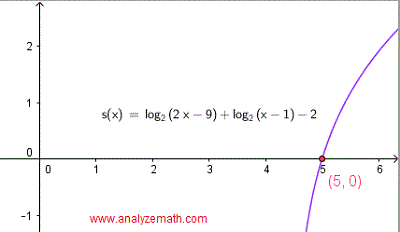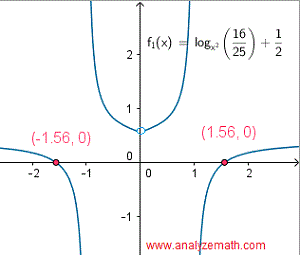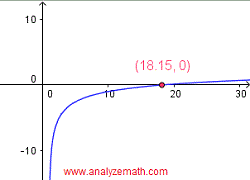# Solve Logarithmic Equations with Solutions for Grade 12

How to Solve Logarithmic Equations? Questions with detailed solutions are presented. The following rules and properties of logarithms are used to solve these equations.

## Properties of Logarithms

Log b A + Log b B = Log b (A B)
Log
b A - Log b B = Log b (A / B)
n Log
b A = Log b A n
If Log
b A = Log b B, then A = B
Also the graphical approximation to the solutions of each equation of the form f(x) = g(x) are shown as the x coordinates of the x intercepts of the graph of the function h(x) = f(x) - g(x). This is done by first writing the equation to solve with its right side equal to zero and then graphing the left side and locating the x intercepts.

## Questions: Solve Logarithmic Equations

### Question 1

Solve the equation: log(2x - 3) = log(3 - x) - 2.

### Solution

Rewrite the equation with the log terms on one side.
log(2x - 3) - log(3 - x) = - 2
Rewrite the equation substituting - 2 by log 10-2
log(2x - 3) - log(3 - x) = log 10
-2
Use the log rule log A - Log B = log (A/B) to rewrite the equation as
log ((2x - 3)/(3 - x)) = log 10
-2
Function log(x) being a one to one function, we can write
(2x - 3)/(3 - x) = 10
-2
Solve the above equation
2x - 3 = (3 - x) / 100
200x - 300 = 3 - x
201x = 303
x = 303 / 201 = 101 / 67 ≈ 1.51
Check the solution found.
left side: log(2(101/67) - 3) = log(1/67) = - log(67)
right side: log(3 - 101 / 67) - 2 = log(100/67) - 2 = log(100) - log(67) - 2 = 2 - log(67) - 2 = - log(67)
The given equation has one solution.
x = 101 / 67 ≈ 1.51
The x intercept of the graph of the function q(x) = log(2x - 3) - log(3 - x) + 2 (the left side of the given equation written with its right side equal to zero) is shown below. Note that the x coordinate of the x intercept is close to the solution obtained analytically above..

### Question 2

Solve the equation: log x - log(x 2 - 1) = - 2 log(x - 1).

### Solution

Use the log rule log A - Log B = log (A/B) to rewrite the left side of the equation as one term and the rule n log(x) = log(xn) to rewrite the right side as the log of a power.
log (x/(x
2 - 1)) = log (x - 1) -2
Function log(x) being a one to one function, we can write
x / (x
2 - 1) = (x - 1) -2
Multiply all terms of the above equation by (x - 1)2 and simplify
(x - 1)
2 (x /(x 2 - 1)) = (x - 1) 2 (x - 1) -2
(x - 1)
2 (x /(x 2 - 1)) = 1
Expand (x - 1)2 and (x2 - 1)) and simplify
x(x - 1)(x - 1) / ((x + 1)(x - 1)) = 1
x(x - 1) / (x + 1) = 1
Multiply both sides of the equation by x + 1 and simplify.
x(x - 1) = x + 1
x
2 - 2 x - 1 = 0
Two solutions: x
1 = 1 + √ 2 ≈ 2.41 and x 2 = 1 - √ 2 ≈ - 0.41
Check the solutions found.
x
1 = 1 + √ 2
left side: log (1 + √ 2) - log((1 + √ 2)
2 - 1) = log (1 + √ 2) - log( 2 + 2 √ 2) = - log(2)
Right side: - 2 log(1 + √ 2 - 1) = -2 log(√ 2) = - log (2)
x
2 = 1 - √ 2
Left side: log (1 - √ 2) - log((1 - √ 2)
2 - 1) is undefined because 1 - √ 2 is negative and the term log (1 - √ 2) is undefined.
The given equation has one solution.
x = 1 + √ 2 ≈ 2.41
The x intercept of the function r(x) = log x - log(x
2 - 1) + 2 log(x - 1) is shown below and its x coordinate is close to the soltuion of the equation..

### Question 3

Solve the equation: log 2 (2x - 9) = 2 - log 2 (x - 1).

### Solution

Rewrite the equation with terms with log on the same side and substitute 2 by log24
log
2 (2x - 9) + log 2 (x - 1) = log 2 4
Use the rule log2A + log2B = log2 (A B) to rewrite the equation as follows
log
2 ( (2x - 9)(x - 1) ) = log 2 4
Which gives
(2x - 9)(x - 1) = 4
Expand and write in standard form.
2 x
2 - 11 x + 5 = 0
Solve the above quadratic equation to obtain
Two solutions: x
1 = 1 / 2 and x 2 = 5
Check the solutions found.
x
1 = 1 / 2
left side: log
2 (2(1/2) - 9) undefined since the argument of the log is negative.
x
2 = 5
Left side: log
2 (2(5) - 9) = 0
Left side: 2 - log
2 (5 - 1) = 2 - log 2 4 = 0
The given equation has one solution.
x = 5
The graphical solution is shown below as the x intercept of the function s(x) = log
2 (2x - 9) - 2 + log 2 (x - 1)..

### Question 4

Solve the equation: $$log_{x^2}(\dfrac{16}{25}) = - 1 / 2.$$

### Solution

Use the inverse relationship between the exponential and logarithmic to the same base to rewrite the equation as:
(x
2 ) -1/2 = 16 / 25
Note that (x2)-1/2 = 1 / (x2)1/2 = 1 / | x | and rewrite the equation as:
1 / | x | = 16 / 25 or | x | = 25 / 16
Which gives the solutions
Two solutions: x
1 = 25/16 and x 2 = -25/16
Check the solutions found.
x
1 = 25/16
left side: log
(25/16)2 (16/25) = log (25/16)2 (25/16) -1 = log (25/16)2 ((25/16) -2 ) 1/2 = log (25/16)2 ((25/16) 2 ) -1/2 = - 1 / 2
x
2 = - 25/16

left side: log
( - 25/16)2 (16/25) = log (25/16)2 (16/25) = - 1 / 2
The given equation has two solution.
x = 25 / 16 and x = - 25 / 16
The graphical solution is shown below as the x intercepts of $$f_1(x) = log_{x^2}(\dfrac{16}{25}) + 1 / 2$$..

### Question 5

Solve the equation: 2 ln(x + 3) - ln(x + 1) = 3 ln 2

### Solution

Use the rules n ln x = ln xn and ln (A/B) = ln A - ln B to rewrite the equation as follows:
ln(x + 3)
2 - ln(x + 1) = ln 2 3
ln ((x + 3)
2 / (x + 1)) = ln 8
ln x is a one to one function, hence
(x + 3)
2 (x + 1) = 8 or (x + 3) 2 = 8(x + 1)
Write the above quadratic equation in standard form.
x
2 - 2 x + 1 = 0
Which gives one solution
One solution: x = 1
Check the solutions found.
left side: 2 ln(1 + 3) - ln(1 + 1) = 2 ln 4 - ln 2 = 4 ln 2 - ln 2 = 3 ln 2
The graphical solution is shown below as the x intercept of h
1 (x) = 2 ln(x + 3) - ln(x + 1) - 3 ln 2..

### Question 6

Solve the equation: (log 2 (x)) 2 - Log 2 (x 2 ) = 8.

### Solution

Use the rules n Log2 x = Log2 xn to rewrite the equation as follows:
(log
2 (x)) 2 - 2 Log 2 (x) = 8
Let u = log2(x) and write the equation in standard form nd in terms of u.
u
2 - 2 u - 8 = 0
Which gives two solutions
One solution: u = -2 and u = 4
We now solve for x.
u = = - 2 = log
2 (x) gives x = 2 -2 = 1/4
u = = 4 = log
2 (x) gives x = 2 4 = 16
The graphical solutions are shown below as the x intercepts of p
1 (x) = (log 2 (x)) 2 - Log 2 (x 2 ) = 8..

### Question 7

Solve the equation: 10 log(log(x)) = 1.

### Solution

Divide both sides by 10
log(log(x)) = 0.1
Which gives
log(x) = 10
0.1
Which gives x as
x = 10
(100.1) ≈ 18.15
We now solve for x.
The graphical solution is shown below of the x intercepts of f(x) = 10 log(log(x)) - 1..